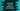# JavaScript array fill method explanation with examples## JavaScript array fill method explanation with examples:

In this post, we will learn how to use the fill() method with explanations. The fill method is used to change the contents of an array to a static value. We can provide the start and end indexes of the array where we want to change the values of the array.

Let’s learn the definition of fill first before moving to examples.

### Definition of fill method:

The fill() method is defined as like below:

``fill(v, s, e)``

Here,

• v is the value to fill in the given array. This is the exact value that will replace the array elements.
• s is the starting index in the array. This is an optional value. If you don’t provide this value, it takes 0 as default. This is an inclusive value.
• e is the end index in the array, which is an exclusive value. This is also optional. By default, it takes the length of the array.

### Return value of fill:

The fill method returns the modified array with the new values.

### Example of fill():

Let me show you one example of fill:

``````const array1 = [1, 2, 3, 4, 5, 6, 7, 8, 9];
const array2 = [1, 2, 3, 4, 5, 6, 7, 8, 9];
const array3 = [1, 2, 3, 4, 5, 6, 7, 8, 9];

console.log(`Example 1: \${array1.fill(0)}`);
console.log(`Example 2: \${array2.fill(0, 2)}`);
console.log(`Example 3: \${array3.fill(0, 2, 5)}`);``````

Here,

• For example 1, it fills 0 for all elements of array1.
• For example 2, it fills 0 from index 2 to the end of the array array2.
• For example 3, it fills 0 from index 2 to index 4 of the array array3.

If you run this program, it will print:

``````Example 1: 0,0,0,0,0,0,0,0,0
Example 2: 1,2,0,0,0,0,0,0,0
Example 3: 1,2,0,0,0,6,7,8,9``````

So, the start and end indexes are optional. If we don’t provide the start index, it will take 0 i.e. the start of the array. Similarly, if we don’t provide the end index, it will take the length of the array by default.

### With negative start and end index values:

Let’s use negative start and end values in the fill method. If we use a negative start index value, it will treat it as length of array + start and similarly if we use a negative end index value, it will treat it as length of array + end.

Let’s try it with an example:

``````const array1 = [1, 2, 3, 4, 5, 6, 7, 8, 9];
const array2 = [1, 2, 3, 4, 5, 6, 7, 8, 9];

console.log(`Example 1: \${array1.fill(0, -2)}`);
console.log(`Example 2: \${array2.fill(0, -4, -1)}`);``````
• The length of the array is 9.
• For the first example, it will take the start index as 9 - 2 or 7.
• For the second example, it will take the start index as 9 - 4 i.e. 5 and end index as 9 - 1 i.e. 8.

It will print the below output:

``````Example 1: 1,2,3,4,5,6,7,0,0
Example 2: 1,2,3,4,5,0,0,0,9``````

### Example of fill with an empty array:

Let’s try to fill an empty array with the fill method:

``````const array1 = [];

console.log(`Example 1: \${array1.fill(0)}`);``````

It will not make any change to the array because it is an empty array.

### How to use Array.fill to populate an empty array:

We can use Array.fill() method to populate an empty array. We can create an empty array of any size and call the fill method to fill it with any value we want.

Let’s check it with an example:

``````const array1 = Array(5).fill(20);

console.log(`array1: \${array1}`);``````

It will create an empty array and fill it with 20. If you run this program, it will print the below output:

As you can see here, it created an array and fill it with 20.